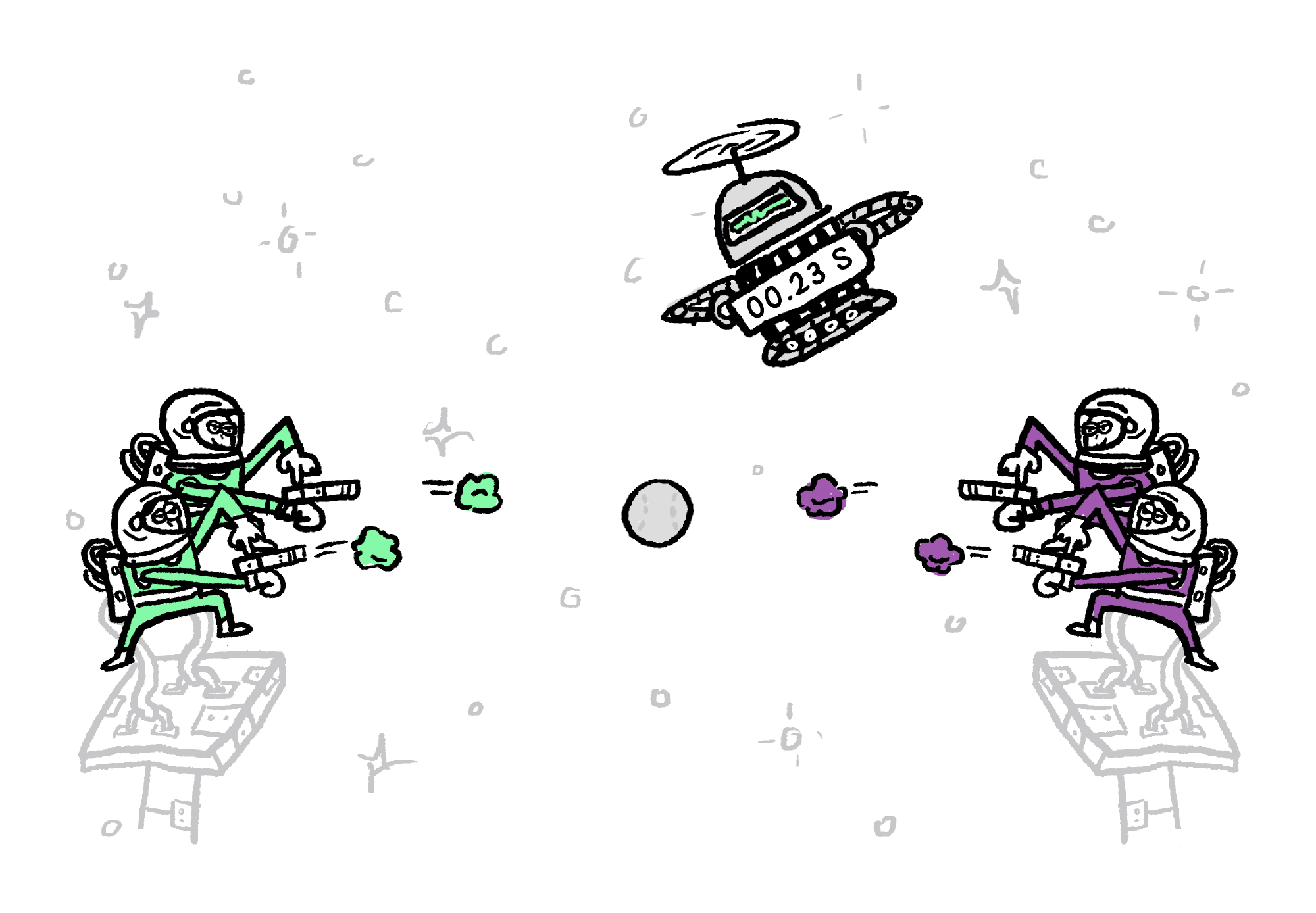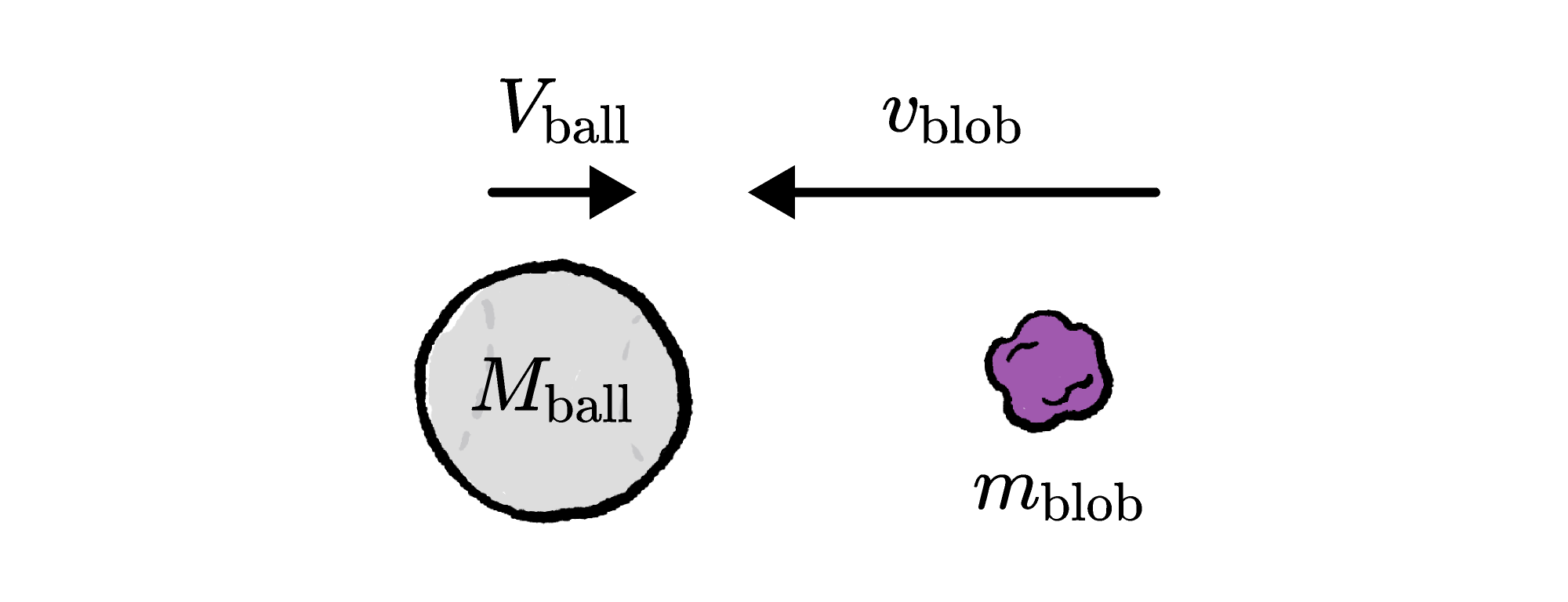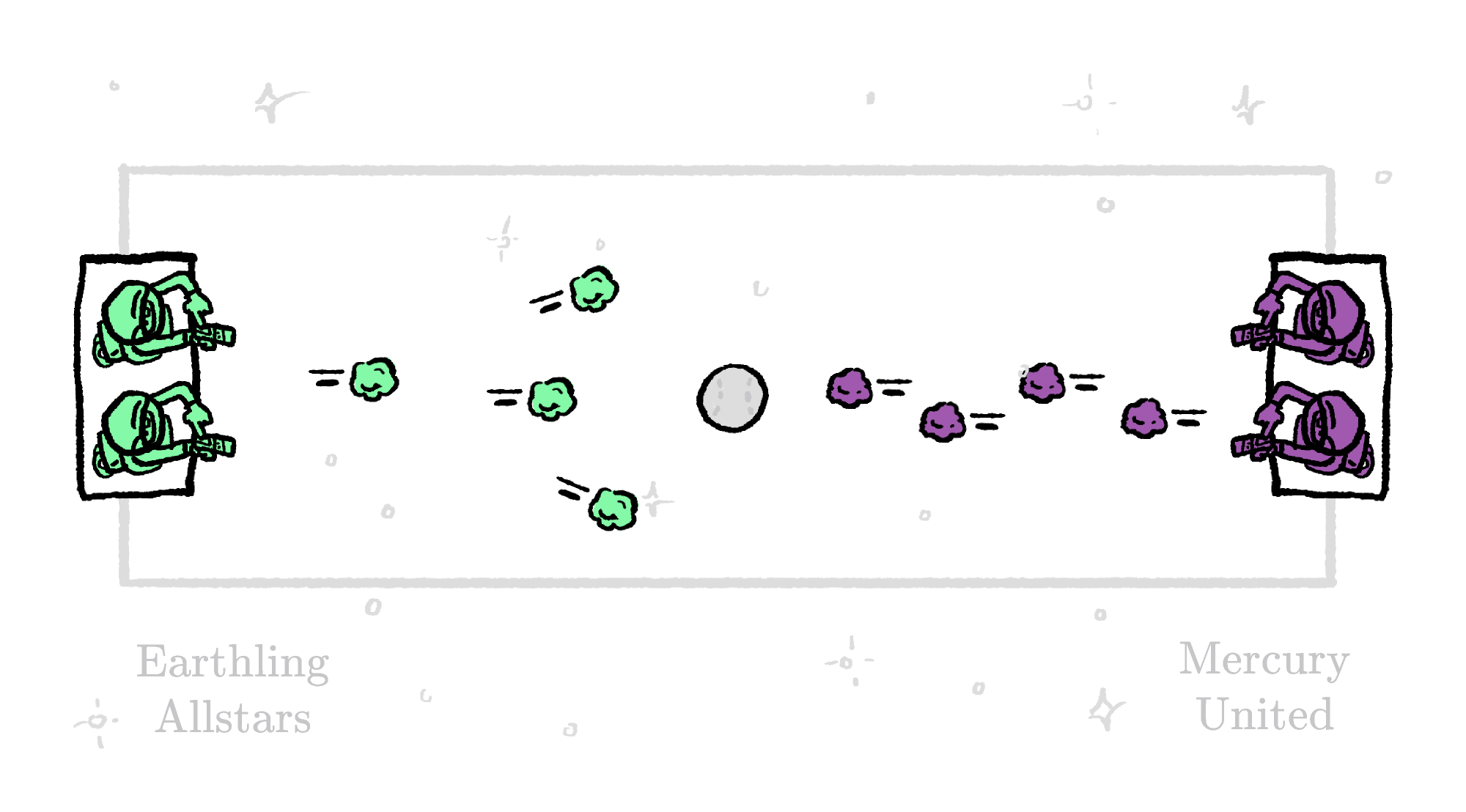Back

## Blaster Ball

Blaster Ball is a deep-space competition in which two teams of astronauts use blaster guns loaded with harmless sticky blobs to knock a ball toward the opposite end of a pitch.Blaster ball pitches are always located out of the range of friction, air resistance, or significant amounts of gravity, which is more convenient for Blaster Ball strategists than for traveling fans. The trick for each team is to transfer as much momentum to the ball as possible with a limited number of shots that must be fired before the game clock runs down.

The momentum of a moving object is its mass times its velocity. When a blob collides with the ball, it loses some of its momentum and the ball's momentum increases by the same amount. This principle can help us find the final speed of the ball and blob.

Before the collision, the ball's velocity is $+V_\text{ball}$ to the right and its mass is $M_\text{ball}.$ The blob is heading to the left with velocity $-v_\text{blob}$ (the minus sign indicates direction). Its mass is $m_\text{blob}.$The total momentum, represented by the symbol $p,$ is

$p_\text{total}=M_\text{ball}V_\text{ball} - m_\text{blob}v_\text{blob}.$

After the blob sticks to the ball, the momentum is still $p_\text{total}.$ The total mass hasn't changed, but the final velocity of the blob and ball (now stuck together) is $V_\text{final},$ so

$p_\text{total}=(M_\text{ball}+m_\text{blob})V_\text{final}.$Setting these expressions for $p_\text{total}$ equal, we can solve for $V_\text{final}:$

\begin{aligned} (M_\text{ball}+m_\text{blob})V_\text{final} &= M_\text{ball}V_\text{ball} - m_\text{blob}v_\text{blob} \\[0.5em] V_\text{final} &= \frac{M_\text{ball}V_\text{ball} - m_\text{blob}v_\text{blob}}{M_\text{ball}+m_\text{blob}}. \end{aligned}

As long as there are no outside forces, the total momentum of all objects involved in a collision is a constant. This useful principle is known as conservation of momentum, and you can extend it to any number of sticky blobs hitting the ball during a round.

The structure of the result above hints at another way of conceptualizing momentum conservation. The right-hand side takes the form of an average: each object's velocity is multiplied (i.e. "weighted") by its respective mass, the products are summed over all objects, and then the sum is divided by the total mass.

This average yields the velocity of the center of mass ("CoM") of the ball and blobs:

$V_\text{CoM}=\frac{\text{mass-weighted sum of velocity}}{\text{total mass}}.$

An equivalent statement of momentum conservation during a collision (or series of collisions) is "the center-of-mass velocity is constant."

If we plot the center of mass along with multiple colliding objects, we can see directly that it moves with a constant velocity as the collisions take place.

Now, if the blobs collide with the ball and stick together, it follows that they must continue moving at the speed of the center of mass. This even works when the colliding objects don't stick together.

The uniform motion of the center of mass in the absence of external forces is one of the best tools in your physicist tool kit for predicting what will happen when things collide, explode, or interact in any way.

# Today's Challenge

In this round of Blaster Ball, the ball weighs $\SI{0.4}{\kilo\gram}$ and each sticky blob weighs $\SI{0.1}{\kilo\gram}.$ The sticky blobs are launched at exactly $\SI[per-mode=symbol]{100}{\meter\per\second}.$ Blobs always stick perfectly to the ball after impact.In the first salvo of the game, the Earthling Allstars only manage $2$ direct hits, while Mercury United scores $4.$ What is the speed of the ball after these collisions?×